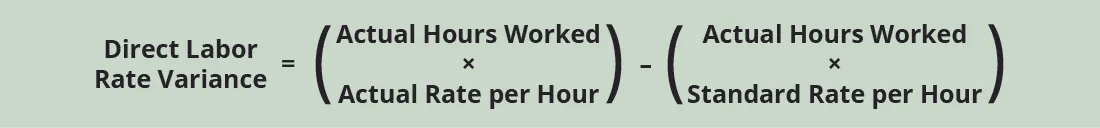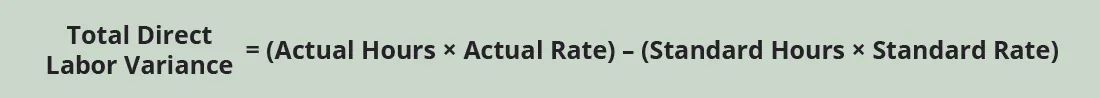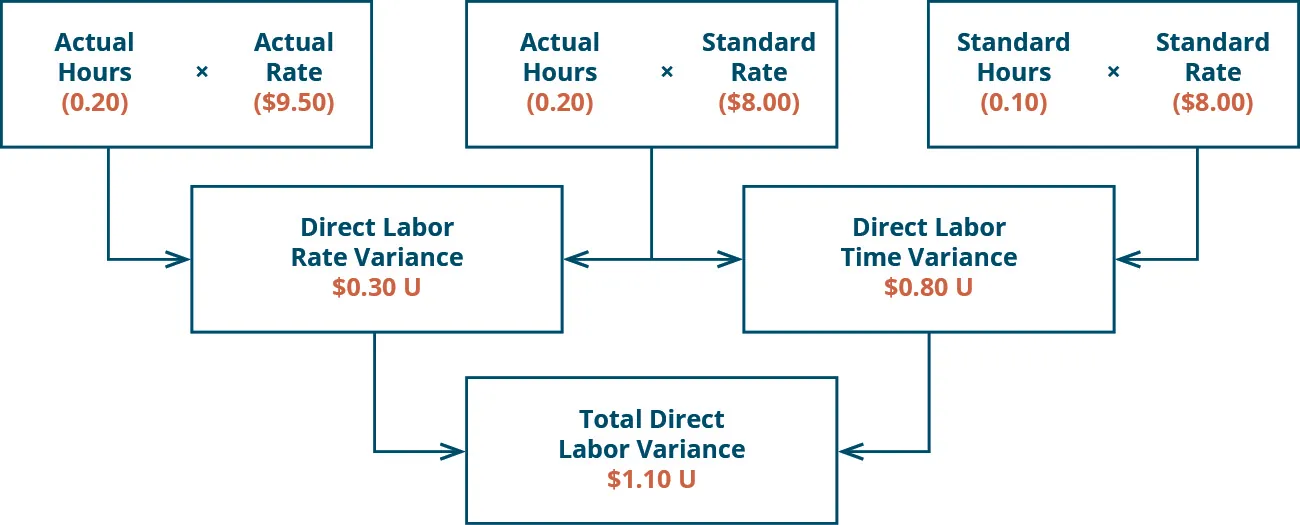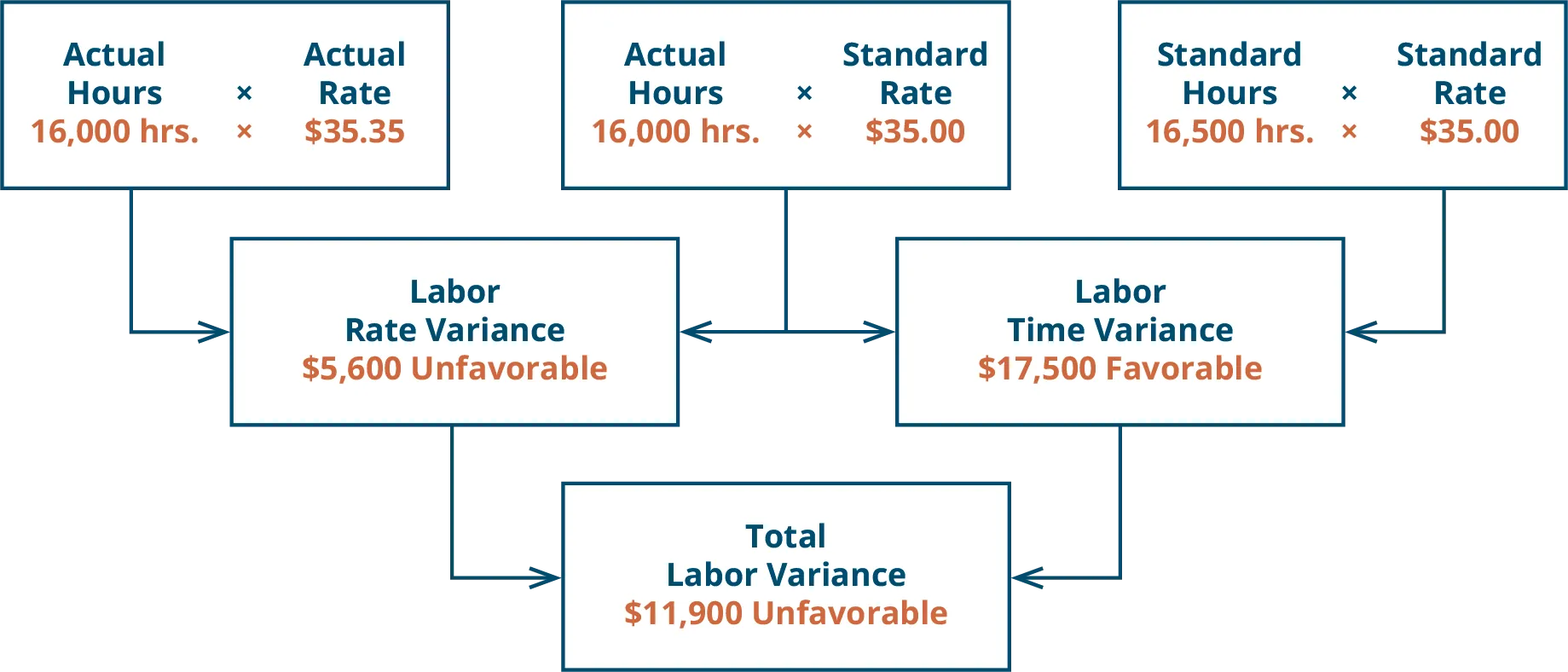Principles of Accounting, Volume 2: Managerial Accounting

# 8.3Compute and Evaluate Labor Variances

Principles of Accounting, Volume 2: Managerial Accounting8.3 Compute and Evaluate Labor Variances

In addition to evaluating materials usage, companies must assess how efficiently and effectively they are using labor in the production of their products. Direct labor is a cost associated with workers working directly in the production process. The company must look at both the quantity of hours used and the rate of the labor and compare outcomes to standard costs. Determining efficiency and effectiveness of labor leads to individual labor variances. A company can compute these labor variances and make informed decisions about labor operations based on these differences.

### Fundamentals of Direct Labor Variances

The direct labor variance measures how efficiently the company uses labor as well as how effective it is at pricing labor. There are two components to a labor variance, the direct labor rate variance and the direct labor time variance.

#### Direct Labor Rate Variance

The direct labor rate variance compares the actual rate per hour of direct labor to the standard rate per hour of labor for the hours worked. The direct labor rate variance is calculated using this formula:Factoring out the actual hours worked from both components of the formula, it can be rewritten asWith either of these formulas, the actual rate per hour refers to the actual rate of pay for workers to create one unit of product. The standard rate per hour is the expected rate of pay for workers to create one unit of product. The actual hours worked are the actual number of hours worked to create one unit of product. If there is no difference between the standard rate and the actual rate, the outcome will be zero, and no variance exists.

If the actual rate of pay per hour is less than the standard rate of pay per hour, the variance will be a favorable variance. A favorable outcome means you paid workers less than anticipated. If, however, the actual rate of pay per hour is greater than the standard rate of pay per hour, the variance will be unfavorable. An unfavorable outcome means you paid workers more than anticipated.

The actual rate can differ from the standard or expected rate because of supply and demand of the workers, increased labor costs due to economic changes or union contracts, or the ability to hire employees at a different skill level. Once the manufacturer makes the products, the labor costs will follow the goods through production, so the company should evaluate how the difference between what it expected to happen and what actually happened will affect all the goods produced using these particular labor rates.

Let us again consider Connie’s Candy Company with respect to labor. Connie’s Candy establishes a standard rate per hour for labor of $8.00. Each box of candy is expected to require 0.10 hours of labor (6 minutes). Connie’s Candy found that the actual rate of pay per hour for labor was$7.50. They still actually required 0.10 hours of labor to make each box. The direct labor rate variance computes as:

$Direct Labor Rate Variance=(7.50–8.00)×0.10hours=–0.05or0.05(Favorable)Direct Labor Rate Variance=(7.50–8.00)×0.10hours=–0.05or0.05(Favorable)$

In this case, the actual rate per hour is $7.50, the standard rate per hour is$8.00, and the actual hour worked is 0.10 hours per box. This computes as a favorable outcome. This is a favorable outcome because the actual rate of pay was less than the standard rate of pay. As a result of this favorable outcome information, the company may consider continuing operations as they exist, or could change future budget projections to reflect higher profit margins, among other things.

Let us take the same example except now the actual rate of pay per hour is $9.50. The direct labor rate variance computes as: $Direct Labor Rate Variance=(9.50–8.00)×0.10hours=0.15or0.15(Unfavorable)Direct Labor Rate Variance=(9.50–8.00)×0.10hours=0.15or0.15(Unfavorable)$ In this case, the actual rate per hour is$9.50, the standard rate per hour is $8.00, and the actual hours worked per box are 0.10 hours. This computes as an unfavorable outcome. This is an unfavorable outcome because the actual rate per hour was more than the standard rate per hour. As a result of this unfavorable outcome information, the company may consider using cheaper labor, changing the production process to be more efficient, or increasing prices to cover labor costs. Another element this company and others must consider is a direct labor time variance. #### Direct Labor Time Variance The direct labor time variance compares the actual labor hours used to the standard labor hours that were expected to be used to make the actual units produced. The variance is calculated using this formula:Factoring out the standard rate per hour from both components of the formula, it can be rewritten as:With either of these formulas, the actual hours worked refers to the actual number of hours used at the actual production output. The standard rate per hour is the expected hourly rate paid to workers. The standard hours are the expected number of hours used at the actual production output. If there is no difference between the actual hours worked and the standard hours, the outcome will be zero, and no variance exists. If the actual hours worked are less than the standard hours at the actual production output level, the variance will be a favorable variance. A favorable outcome means you used fewer hours than anticipated to make the actual number of production units. If, however, the actual hours worked are greater than the standard hours at the actual production output level, the variance will be unfavorable. An unfavorable outcome means you used more hours than anticipated to make the actual number of production units. The actual hours used can differ from the standard hours because of improved efficiencies in production, carelessness or inefficiencies in production, or poor estimation when creating the standard usage. Consider the previous example with Connie’s Candy Company. Connie’s Candy establishes a standard rate per hour for labor of$8.00. Each box of candy is expected to require 0.10 hours of labor (6 minutes). Connie’s Candy found that the actual hours worked per box were 0.05 hours (3 minutes). The actual rate per hour for labor remained at $8.00 to make each box. The direct labor time variance computes as: $Direct Labor Time Variance=(0.05–0.10)×8.00per hour=–0.40or0.40(Favorable)Direct Labor Time Variance=(0.05–0.10)×8.00per hour=–0.40or0.40(Favorable)$ In this case, the actual hours worked are 0.05 per box, the standard hours are 0.10 per box, and the standard rate per hour is$8.00. This computes as a favorable outcome. This is a favorable outcome because the actual hours worked were less than the standard hours expected. As a result of this favorable outcome information, the company may consider continuing operations as they exist, or could change future budget projections to reflect higher profit margins, among other things.

Let us take the same example except now the actual hours worked are 0.20 hours per box. The direct labor time variance computes as:

$Direct Labor Time Variance=(0.20–0.10)×8.00per hour=0.80or0.80(Unfavorable)Direct Labor Time Variance=(0.20–0.10)×8.00per hour=0.80or0.80(Unfavorable)$

In this case, the actual hours worked per box are 0.20, the standard hours per box are 0.10, and the standard rate per hour is $8.00. This computes as an unfavorable outcome. This is an unfavorable outcome because the actual hours worked were more than the standard hours expected per box. As a result of this unfavorable outcome information, the company may consider retraining its workers, changing the production process to be more efficient, or increasing prices to cover labor costs. The combination of the two variances can produce one overall total direct labor cost variance. ### Think It Through #### Package Deliveries UPS drivers are evaluated on how many miles they drive and how quickly they deliver packages. The drivers are given the route and time they are expected to take, so they are expected to complete their route in a timely and efficient manner. They also work until all packages are delivered. A GPS tracking system tracks the trucks throughout the day. The system keeps track of how much they back up and if they take any left turns because right turns are much more time efficient.3 Tracking drivers like this does not leave them very much time to deal with customers. Customer service is a major part of the driver’s job. Can the driver service the customer and drive the route in the time and distance allotted? Which is more important: customer service or driving the route in a timely and efficient manner? #### Total Direct Labor Variance When a company makes a product and compares the actual labor cost to the standard labor cost, the result is the total direct labor variance.If the outcome is unfavorable, the actual costs related to labor were more than the expected (standard) costs. If the outcome is favorable, the actual costs related to labor are less than the expected (standard) costs. The total direct labor variance is also found by combining the direct labor rate variance and the direct labor time variance. By showing the total direct labor variance as the sum of the two components, management can better analyze the two variances and enhance decision-making. Figure 8.4 shows the connection between the direct labor rate variance and direct labor time variance to total direct labor variance. Figure 8.4 Direct Labor Variance. (attribution: Copyright Rice University, OpenStax, under CC BY-NC-SA 4.0 license) For example, Connie’s Candy Company expects to pay a rate of$8.00 per hour for labor but actually pays $9.50 per hour. The company expected to use 0.10 hours of labor per box but actually used 0.20 hours per box. The total direct labor variance is computed as: $Total Direct Labor Time Variance=(0.20hours×9.50)–(0.10hours×8.00)=1.90–0.80=1.10(Unfavorable)Total Direct Labor Time Variance=(0.20hours×9.50)–(0.10hours×8.00)=1.90–0.80=1.10(Unfavorable)$ In this case, two elements are contributing to the unfavorable outcome. Connie’s Candy paid$1.50 per hour more for labor than expected and used 0.10 hours more than expected to make one box of candy. The same calculation is shown as follows using the outcomes of the direct labor rate and time variances.As with the interpretations for the labor rate and time variances, the company would review the individual components contributing to the overall unfavorable outcome for the total direct labor variance, and possibly make changes to production elements as a result.

#### Sweet and Fresh Shampoo Labor

Biglow Company makes a hair shampoo called Sweet and Fresh. Each bottle has a standard labor cost of 1.5 hours at $35.00 per hour. During May, Biglow manufactured 11,000 bottles. They used 16,000 hours at a cost of$565,600. Calculate the labor rate variance, labor time variance, and total labor variance.

Solution### Concepts In Practice

#### Labor Costs in Service Industries

In the service industry, labor is the main cost. Doctors, for example, have a time allotment for a physical exam and base their fee on the expected time. Insurance companies pay doctors according to a set schedule, so they set the labor standard. They pay a set rate for a physical exam, no matter how long it takes. If the exam takes longer than expected, the doctor is not compensated for that extra time. This would produce an unfavorable labor variance for the doctor. Doctors know the standard and try to schedule accordingly so a variance does not exist. If anything, they try to produce a favorable variance by seeing more patients in a quicker time frame to maximize their compensation potential.

### Footnotes

• 3Graham Kendall. “Why UPS Drivers Don’t Turn Left and Why You Shouldn’t Either.” The Conversation. January 20, 2017. http://theconversation.com/why-ups-drivers-dont-turn-left-and-you-probably-shouldnt-either-71432
Order a print copy

As an Amazon Associate we earn from qualifying purchases.